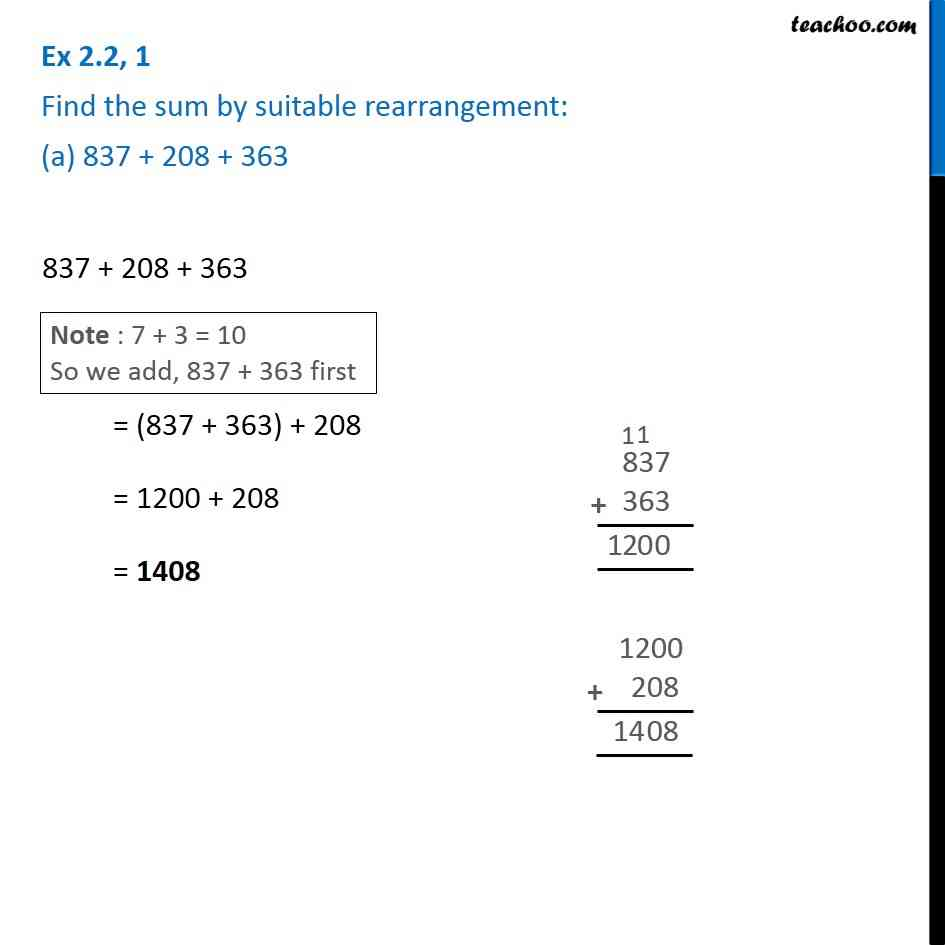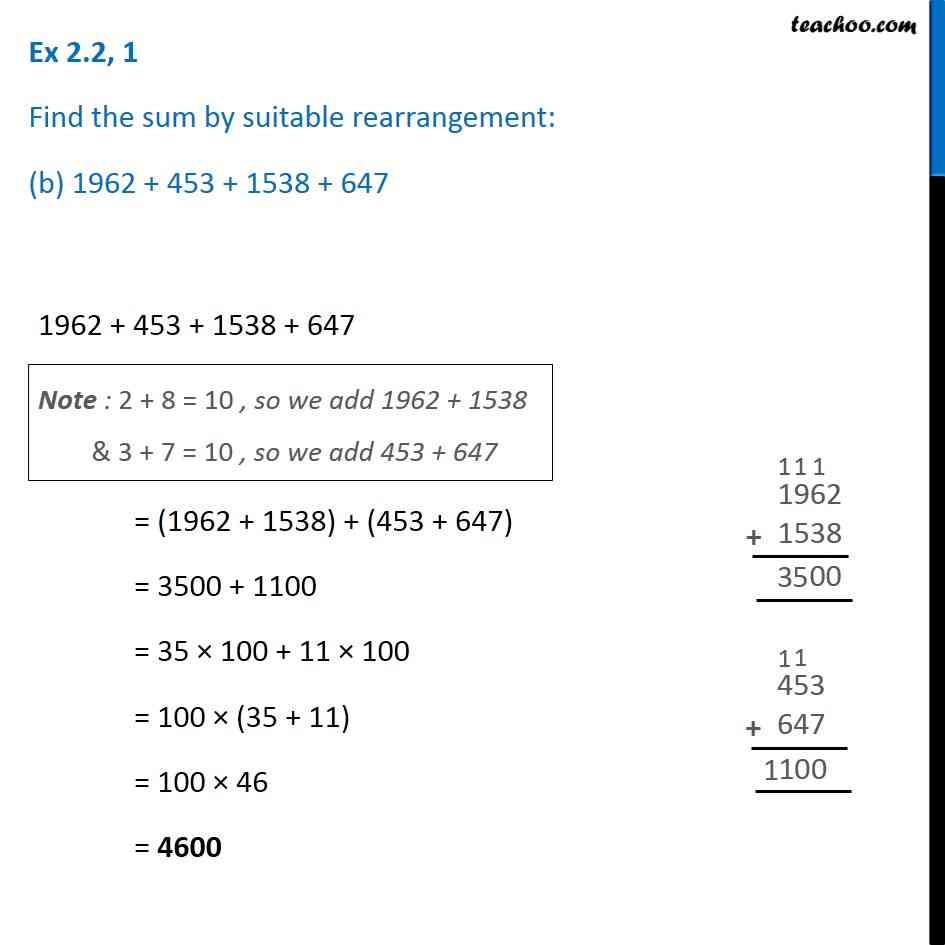1. Chapter 2 Class 6 Whole Numbers
2. Serial order wise
3. Ex 2.2

Transcript

Ex 2.2, 1 Find the sum by suitable rearrangement: (a) 837 + 208 + 363 837 + 208 + 363 = (837 + 363) + 208 = 1200 + 208 = 1408 Ex 2.2, 1 Find the sum by suitable rearrangement: (b) 1962 + 453 + 1538 + 647 1962 + 453 + 1538 + 647 = (1962 + 1538) + (453 + 647) = 3500 + 1100 = 35 × 100 + 11 × 100 = 100 × (35 + 11) = 100 × 46 = 4600

Ex 2.2